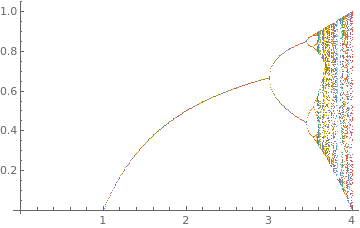# Preface

This secton is devoting to discrete logostoc equation.

# Discrete logistic recurrence

The logistic map is a polynomial mapping (equivalently, recurrence relation) of degree 2) that is describd by the recurrence:

$x_{n+1} = r\,x_n \left( 1- x_n \right) , \qquad n=0,1,2,\ldots .$
The map was popularized by the biologist Robert May in 1976 paper. In part as a discrete-time demographic model analogous to the logistic equation first created by Pierre François Verhulst. As it is usually the case, a discrete analoug of a continuous model has some feature that are not present in its continuous counterpart. It turns out that the dicrete logistic equation has many properties that do not belong to the corresponding differential equation.
f[r_][x_] := r*x*(1 - x) // N
t1 = Table[{r, Nest[f[r], .5, n]}, {r, 2.8, 4.1, 1.2/249}, {n, 101, 300}];
toshow = Flatten[t1, 1];
ListPlot[toshow, PlotRange -> {{3.7, 4}, {0; 1}}, PlotStyle -> PointSize[0.004]]Output of discrete logistic recurrence Mandelbrot setDiscrete logistic recurrence: ListPlot[Table[ Thread[{r, Nest[r # (1 - #) &, Range[0, 1, 0.01], 1000]}], {r, 0, 4, 0.01}]] Solutions of discrete logistic recurrence. Mathematica code

Example: Using real data and simply perform the least squares best fit of a quadratic function (without the constant term) through this data, we obtain the logistic grow model:

$P_{n+1} = 1.351\, P_n - 1.232 \times 10^{-9} P_n^2 , \qquad n=0,1,2,\ldots .$

Hénon map

The Hénon map, sometimes called Hénon-Pomeau attractor/map, is a discrete-time dynamical system. It is one of the most studied examples of dynamical systems that exhibit chaotic behavior. The Hénon map takes a point (xn, yn) in the plane and maps it to a new point
$\begin{cases} x_{n+1} &= 1 - a\,x_n^2 + y_n \\ y_{n+1} &= b\,x_n . \end{cases}$
The map depends on two parameters, 𝑎 and b, which for the classical Hénon map have values of 𝑎 = 1.4 and b = 0.3. For the classical values the Hénon map is chaotic. For other values of a and b the map may be chaotic, intermittent, or converge to a periodic orbit. An overview of the type of behavior of the map at different parameter values may be obtained from its orbit diagram.

The map was introduced by Michel Hénon (1931--2013) as a simplified model of the Poincaré section of the Lorenz model. For the classical map, an initial point of the plane will either approach a set of points known as the Hénon strange attractor, or diverge to infinity. The Hénon attractor is a fractal, smooth in one direction and a Cantor set in another. As an example, consider the Standard Map:

$\begin{cases} x_{n+1} &= \left( x_n + y_n \right) \mod 2\pi \\ y_{n+1} &= \left[ y_n + b\,\sin (x_n ) \right] \mod 2\pi . \end{cases}$
The Standard Map is a generic description for a physical system with periodic motion which is perturbed by an external oscillating force. Examples are a pendulum which is periodically pushed, or an electron in a magnetic field (which forces it into a circular or helical orbit), influenced by an oscillating electrostatic field. The x and y coordinates represent the so-called action and angle variables of such physical systems, which decsribe the orbit of the electron (or the tip of the pendulum) in phase space. The Standard Map is a conservative mapping, meaning that a volume in phase space does not contract or expand along its orbit (its shape, however, may be distorted dramatically). This corresponds to closed physical systems in which energy is conserved.

Here b is the stochasticty parameter. For b = 0, the system corresponds for example to the ideal pendulum, exhibiting a completly regular motion. For b > 0, the pendulum is disturbed by a periodic driving force. This has the dramatic effect of producing stochastic orbits for certain initial conditions (i.e. points in phase space). The standard treatment of physics is to deal with the perturbation by expanding the driving force using a Taylor series and to keep only the linear part, with the understanding that higher order contributions are sufficiently small to be neglected. However, this treatment completely misses the qualitative aspects of the driven pendulum. With increasing b increasingly large fractions of phase space become chaotic. Chaotic orbits extend over a two-dimensional area in the above mapping plane. In addition, there are periodic orbits, indicated by a finite number of points on the mapping plane, and quasi-periodic orbits, which produce one or more closed lines (loops) in the mapping plane. Note that for conservative mappings, regular and chaotic phase space regions are arranged on many scales in a self-similar fashion, giving a strong indication that this structure is fractal.

1. Chirikov, B. V. "A Universal Instability of Many-Dimensional Oscillator Systems." Phys. Rep. 52, 264-379, 1979.
2. Cvitanović, P., Gunaratne, G., Procaccia, I., (1988). "Topological and metric properties of Hénon-type strange attractors". Physical Review A. 38 (3): 1503–1520. doi:10.1103/PhysRevA.38.1503.
3. M. Hénon (1976). "A two-dimensional mapping with a strange attractor". Communications in Mathematical Physics. 50 (1): 69–77. doi:10.1007/BF01608556.
4. Michel Hénon and Yves Pomeau (1976). "Two strange attractors with a simple structure,". Turbulence and Navier Stokes Equations. Springer: 29–68
5. Luhn, A. Another interactive iteration of the Hénon Map.
6. MacKay, R. S. and Percival, I. C. "Converse KAM: Theory and Practice." Comm. Math. Phys. 98, 469-512, 1985.